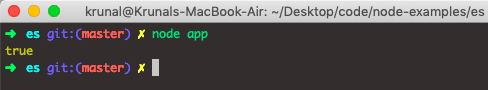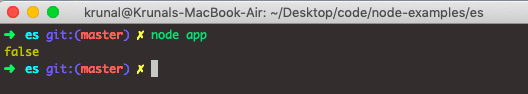# JavaScript Array every() Method

JavaScript Array every() is an instance method that tests whether all elements in an array pass a provided test or condition. The method iterates through each array element and applies a callback function. If the callback function returns a truthy value for every element, the every() method returns true. The method returns false if the callback function returns a false value for at least one element.

### Syntax

``array.every(function(currentValue, index, arr), thisValue)``

### Parameters

The callback function is invoked with three arguments: a value of the element, an element index, and the Array object being traversed.

currentValue: It is required, and the value of the current element.

index: It is Optional. The array index of the current element.

arr: Optional. The array object to the current element belongs.

thisValue: Optional. A value is to be passed to the function to be used as its “this” value. If this parameter is empty, the value “undefined” will be passed as its “this” value.

If a thisValue parameter is provided to every, it will be used as a callback’s this value. Otherwise, the value undefined will be used as this value.

### Example 1

``````let arr = [1, 11, 21, 31, 41]

const diff = (currentValue) => currentValue < 51

console.log(arr.every(diff))``````

In the above example, the array contains the five elements.

Now, in the javascript array, every function takes one callback function. The callback function is a diff function, which takes the currentValue of the array and checks the condition, which is < 51.

If all the elements are under 51, it returns true. Otherwise, it will give us a false output. Here, also we have used the arrow function.

Output### Example 2

Let’s change one element of an array to 61 and test the output.

``````let arr = [1, 11, 21, 31, 61]

const diff = (currentValue) => currentValue < 51

console.log(arr.every(diff))``````

So, here the last element of an array is higher than 51. So, the output will be false.That’s it for this tutorial.

This site uses Akismet to reduce spam. Learn how your comment data is processed.